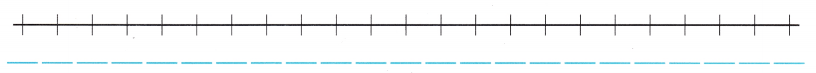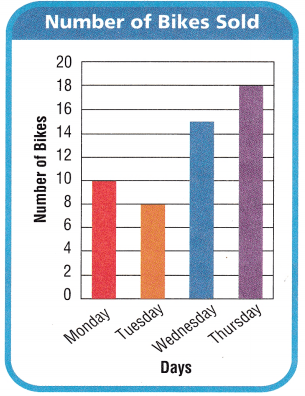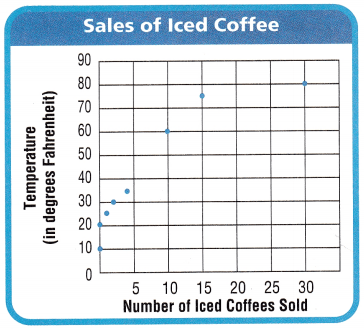Refer to our Texas Go Math Grade 5 Answer Key Pdf to score good marks in the exams. Test yourself by practicing the problems from Texas Go Math Grade 5 Unit 5 Assessment Answer Key.

Vocabulary

Choose the best term from the box.

Question 1.
____ is the number of times an event occurs, (p. 545)
Answer: Experimental probability is the number of times an event occurs.

Question 2.
In a ____ place value, the data is arranged by place value. (p. 583)
Answer: In a Indian place value, the data is arranged by place value

Concepts and Skills

Use the data for 3-6.Question 3.
Make a dot plot to show the data. TEKS 5.9.AQuestion 4.
Use the dot plot to find how many students scored more than 95 points on the test. TEKS 5.9.C
By using the Dot plot 2 students have scored more than 95 points in the test.

Question 5.
Show the data in a stem-and-leaf plot. TEKS 5.9.AQuestion 6.
Use the stem-and-leaf plot to find the range of scores on the math test. TEK 5.9.C.

Use the bar graph for 7-9.

Question 7.
How many more bikes were sold on Monday and Tuesday combined than on Wednesday? TEKS 5.9.C
(A) 15
(B) 3
(C) 5
(D) 7
Explanation:
Number of bikes sold on Monday and Tuesday combined is 18
Number of bikes sold on Wednesday is 15
18 – 15 = 3Question 8.
Four fewer bikes were sold on Wednesday than on Friday. How many bikes were sold on Friday? TEKS 5.9.C
(A) 15
(B) 11
(C) 19
(D) 20
Explanation:
Four fewer bikes were sold on Wednesday than on Friday
15 + 4 = 19
On Friday 19 bikes were sold

Question 9.
The bike shop owner wants to add another bar to the graph to show the number of bikes sold on Saturday. If the number of bikes sold on Saturday is greater than Wednesday but fewer than Thursday, which of the following could be the height of the bar? TEKS 5.9.A
(A) 14
(B) 20
(C) 17
(D) 33
(C) 17
Explanation:
The bikes sold on Saturday is 17
Number of bikes sold on Saturday is greater than Wednesday but fewer than Thursday,
Wednesday is 15
Thursday is 18

Question 10.
The table shows the time it takes students to complete a math test in hours. How many dots should be placed in a dot plot to show the times greater than 1$$\frac{1}{2}$$ hours? TEKS 5.9.A(A) 5
(B) 6
(C) 4
(D) 1

Texas Test Prep

Use the data and frequency table for 11-12.

Question 11.
Chris uses the data to create a frequency table to show the number of bird sightings on a bird watching trip. Which number belongs in the frequency table for tern sightings? TEKS 5.9.AQuestion 12.
How many more gulls than egrets does Chris see? TEKS5.9.C
(A) 2
(B) 5
(C) 3
(D) 7Explanation:
There are 3 gulls more than egret
Number of gulls is 5
Number of egret is 2
5 – 2 = 3

Use the scatter plot for 13-14.

Question 13.
How many iced coffees would you predict will be sold when the temperature is 70° Fahrenheit? TEKS 5.9.C